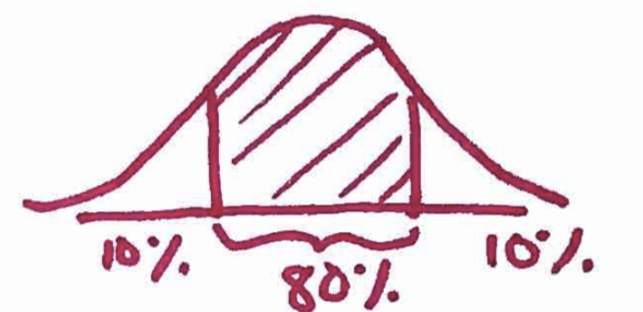## Day 81 - Lesson 8.2

##### Learning Targets
• State and check the Random, 10%, and Large Counts conditions for constructing a confidence interval for a population proportion.

• Determine the critical value for calculating a C% confidence interval for a population proportion using a table or technology.

• Construct and interpret a confidence interval for a population proportion.

##### Activity:

This is always one of the best Activities of the year! Students are trying to figure out the proportion of Hershey’s Kisses that will land flat when dropped onto a table. This Activity is also exciting because students are able to put together their learning from the past few chapters into one goal: constructing a confidence interval.

This Activity is a bit longer than others, so students need to work efficiently. We suggest taking the students through question #8 as a whole group in order to avoid a common student error (see below).

##### The Story of Inference - Making Connections

In Chapter 2, students learned about the 68-95-99.7 rule for Normal Distributions. They learned that 95% of the data in a Normal Distribution is within 2 standard deviations of the mean (yes, we lied to them…we know better now to use 1.96). This idea becomes the critical value for a confidence interval.

In Chapter 4, students learned that the purpose of a random sample is so that we can generalize to the larger population. In this chapter, this idea becomes the Random condition for inference.

In Chapter 6, students learned to check the 10% condition in the binomial setting to be sure that there is independence between trials. In Chapter 7, students used the 10% condition to be sure they could use certain formulas for the standard deviation of sampling distributions.  In this chapter, this idea becomes the 10% condition for inference.

In Chapter 6, students learned to check the Large Counts condition in the binomial setting to be sure that the binomial distribution could be modeled with a Normal distribution. In Chapter 7, students extended this reasoning to apply to the sampling distribution of a sample proportion. In this chapter, this idea becomes the Large Counts condition for inference.

##### Common Student Error

When calculating critical values from Table A, students will mistakenly look for the wrong area. For example, when finding the critical value for an 80% confidence interval, naturally students want to look for an area of 0.80. But we know that Table A gives the area to the left of a specific z-score, which includes the 10% area in the left tail. So students should be looking for an area of 0.90. This will only matter for a few days, because once students get familiar with Table B (take a look at the last row), they will no longer want to use Table A for critical values.